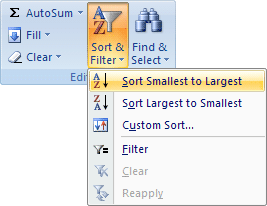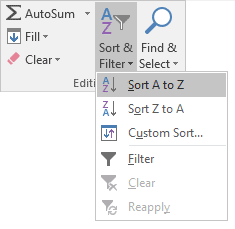# The Excel LOOKUP Function

The LOOKUP function in Excel is used to search one column of data and find data in the corresponding row. For example, if you are searching a column of employee IDs the LOOKUP function can find, say, employee number 12345 in the ID column. Once it has found the ID 12345 it then can return data from that same row. Let's create a spreadsheet to clear things up.

(The LOOKUP function we'll examine is called a VECTOR LOOKUP. There's also an ARRAY LOOKUP, but we won't cover that here.)So we have item numbers in the A column, then a list of products in the B column. Prices are in the C column. Our spreadsheet only has 10 items, but it could have hundreds, even thousands of entries. What we'd like to do is to take an item number and see what Shoe Type, or what Price corresponds to that item number. An item number of 229, for example, would return either "Stacked heel" or a price of 70. We can use the LOOKUP function for this.

The LOOKUP function needs three between its round brackets. It needs these:

LOOKUP(value_to_lookup, data_to_search, results_column)

The value to lookup would be 229 in our example. This is in the A column. So the data to search is A2 to A10. If we want the results to come from the B column, we need the data from B2 to B10. If we wanted the Price to be returned, the data would be in C2 to C10.

So we can add the following LOOKUP function to return a Shoe Type:

=LOOKUP(229, A2:A10, B2:B10)

Or this one, for the Price:

=LOOKUP(229, A2:A10, C2:C10)

So click into cell F1 in your spreadsheet. Click inside the formula bar and enter:

=LOOKUP(229, A2:A10, B2:B10)

When you've entered the formula, press Enter. You'll immediately see that there is a problem:Cell F1 has #N/A in it, indicating there the value is not available. The reason for this error is that LOOKUP needs the data you're searching to be sorted, otherwise problems like this will occur.

To sort the data, highlight the cells A2 to C10. With the cells highlighted, click the Editing panel on the Home ribbon. From the Editing panel, click Sort & Filter. Then select Smallest to Largest from the menu:When your data is sorted, LOOKUP should produce the correct result:So the Shoe Type for item number 229 is Stacked heel.

There are some things to be aware of with the LOOKUP function. If the item you're searching for is less than the smallest item in your data then an error will occur. As an example, try entering 100 as the first number in your LOOKUP function:

=LOOKUP(100, A2:A10, B2:B10)

The N/A error should appear in cell F1 again.

If LOOKUP can't find your value then it will return the highest value lower than your number. So for us, the highest value lower than 229 is 156.

As well as entering a number like 229 you can change it to a cell reference. So the formula could be this:

=LOOKUP(F1, A2:A10, B2:B10)

In cell F1 you'd enter the value you want to search for.

The value you're searching for doesn't have to be a number. You can enter text as well. For example, suppose we wanted to return the item number for Mules. We'd do it like this:

=LOOKUP("Mules", B2:B10, A2:A10)

The value to search for is now text, surrounded by double quotes. For us, the text is in the B column, B2 to B10. The Item Number is in the A column, A2 to A10. Excel will search for the text "Mules" in the B column and return the Item Number from the A column. (Instead of typing Mules in double quotes you can change it to a cell reference, if you prefer.)

In the next section, we'll take a look at VLOOKUP.

<--Back to the Excel Contents Page